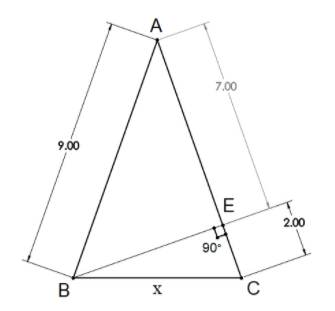QuestionAnswers

# In the following figure of an isosceles triangle, find the value of $'x'$?(All dimensions are in centimeter)Hint: Notice that there are two right-angled triangles in the triangle $\Delta ABC$. They have a common side. Use Pythagoras’ theorem to find the measure of that side. Then use that value while applying Pythagoras’ theorem in another triangle to obtain the value of $'x'$.

Let’s analyse the question through the diagram first. Here we have an isosceles triangle $\Delta ABC$, which has an altitude $BE$ on the side $AC$. The altitude divides the $\Delta ABC$ into two right-angled triangles $\Delta BEC$ and $\Delta BEA$. Using all this information we need to find the value of $'x'$
If we notice $\Delta BEC$ in the figure, we can see that $x$ is the hypotenuse of this triangle.
Now we can use Pythagoras’ theorem that states that, “the sum of the squares of the sides of a right triangle is equal to the square on the hypotenuse”
Using Pythagoras’ theorem in $\Delta BEA$, we get:
$\Rightarrow A{B^2} = B{E^2} + A{E^2}$
Now we can easily substitute the given values in the above equation
$\Rightarrow {9^2} = B{E^2} + {7^2}$
$\Rightarrow B{E^2} = 81 - 49 = 32$
$\Rightarrow BE = \sqrt {32} = 4\sqrt 2 cm$
Similarly, again using Pythagoras’ theorem in $\Delta BEC$, we can see that:
$\Rightarrow B{C^2} = B{E^2} + E{C^2}$
Let’s substitute the already known values in the above equation
$\Rightarrow B{C^2} = {\left( {4\sqrt 2 } \right)^2} + {2^2} = 32 + 4 = 36$
$\Rightarrow BC = x = 6 cm$
Hence, we got the value of $'x'$ as $6 cm$.

Note: Try to first understand all the given information in the diagram given in the question. Be careful while taking square root or squaring both sides in equations. An alternative approach to the problem is to apply Pythagoras’ theorem in both the right-angled triangles and equate for the value of $BE$. This will give you an equation with one unknown, i.e. $'x'$.